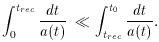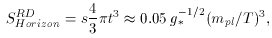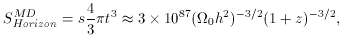A. The Horizon Problem

Why is the universe so homogeneous and isotropic on large scales? Radiation on opposite sides of the observable universe today appear uniform in temperature. Yet, there was not enough time in the past for the photons to communicate their temperature to the opposing sides of the visible universe (i.e., establish thermal equilibrium). Consider the comoving radius of the causally connected parts of the universe at the time of recombination compared to the comoving radius at the present, found from Equation (6) (remember c == 1).(42)

This means a much larger portion of the universe is visible today, than was visible at recombination when the CBR was `released'. So the paradox is how the CBR became homogeneous to 1 part in 105 as we discussed in Part II. There was no time for thermal equilibrium to be reached. In fact, any region separated by more than 2 degrees in the sky today would have been causally disconnected at the time of decoupling .

This argument can be made a bit more quantitative by consideration of the entropy, S, which indicates the number of states within the model. This can be used as a measure of the size of the particle horizon .(43)(44)

where mpl is the Planck mass, s is the entropy density, g* is the particle degeneracy, and z is the redshift. These equations for the entropy of the horizon in a radiation dominated (43) and matter dominated universe (44), are presented only to motivate the following estimates. For an explanation please consult .

At the time of recombination (z1100), when the universe was matter dominated, equation (44) gives a value of about 1083 states. Compared with a value today of 1088 states, this is different by a factor of 105. Thus, there are approximately 105 causally disconnected regions to be accounted for in the observable universe today. The hot Big Bang offers no resolution for this paradox, especially since it is assumed to be an adiabatic (constant entropy) expansion.